CLAT  >  Mixture And Alligation

# Mixture And Alligation - Notes | Study Quantitative Techniques for CLAT - CLAT

 Table of contentsRule of AlligationPractice Question1 Crore+ students have signed up on EduRev. Have you?

• Mixture: The new product obtained by mixing two or more ingredients in a certain ratio is called a mixture of those particular ingredients.
• Alligation: It is a method of solving arithmetic problems related to mixtures of ingredients. This rule enables us to find the ratio in which two or more ingredients at the given price must be mixed to produce a mixture of the desired price.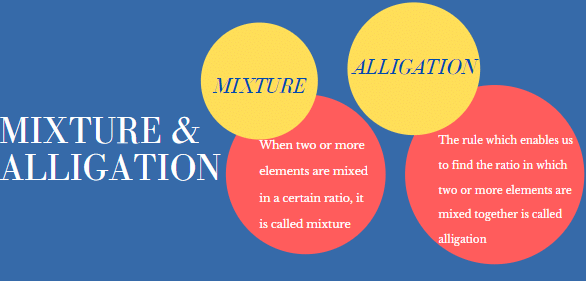Rule of Alligation

Alligation is a rule that enables us to find the ratio in which two or more ingredients at the given price must be mixed to produce a mixture of the desired price.
There are two types of methods used in alligation.

(a) Alligation Method 1

The rule also helps to find out the mean or average value of mixture when the prices of two or more ingredients which may be mixed together and the ratio in which they are mixed are given.
When two ingredients at given prices are known, then the ratio in which these two are mixed to obtain a mixture of known price is given by: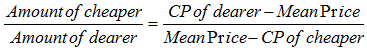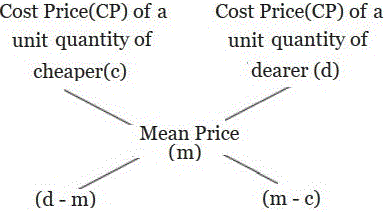Hence, Amount of Cheaper: Amount of dearer = (D – M) : (M – C)

Note:
(1) This method can be used when per cent, per hour, per km, per kg etc are being compared
(2) The CP of the unit quantity of the mixture is called the mean price (M)

Example 1: In what ratio, wheat at Rs 6.20 per kg be mixed with wheat at Rs 7.20 per kg, so that the mixture is worth Rs 6.50 per kg?

Sol.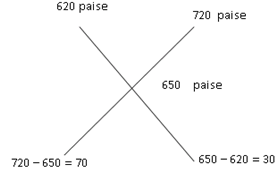Required  ratio is 70 : 30 or 7 : 3

Example 2: In what ratio Rice worth Rs 30 per kg should be mixed with rice worth Rs 32.5 per kg so that on selling the mixture at Rs 34.10 per kg, the profit is 10%.

Sol. Cost price of rice so that the profit is 10% when SP is 34.10 = 34.10 x (100/110) = Rs 31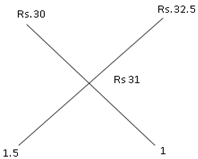The ratio in which rice is to be mixed is 1.5:1 Or 3:2

Example 3: The mixture of a certain quantity of milk with 16 litres of water is worth 0.75 per litre. If pure milk is worth 2.25 per litre, how much milk is there in the mixture?

Sol. Cost of water is Rs 0 per litre.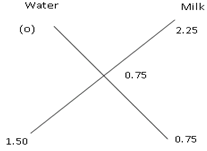Water : Milk = 1.50 : 0.75 = 2 : 1

⇒ Quantity of milk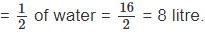(b) Alligation Method 2: Repeated Dilution

This is used to calculate pure quantity left after 'n' number of processes of repeated replacement is done on the pure quantity. Suppose, a container contains 'x' units of a liquid from which 'y' units are taken out and replaced by water.
After 'n' operations quantity of pure will be: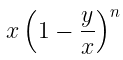Example 4: A container contains 50 litres of milk. From this container, 10 litres of milk was taken out and replaced by water. This process is repeated one more time.

How much milk is now left in the container?

Sol. Units of milk in the container, x = 50 L
Units of milk to be replaced, y = 10 L
number of process of repeated replacement = 2

Applying the Replacement Method: Amount of milk after 2 operations =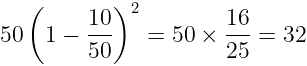Practice Question

Question for Mixture And Alligation
Try yourself:A container contains 40 liters of milk. From this container, 4 liters of milk were taken out and replaced by water. This process was repeated further two times. How much milk is now contained by the container?

Question for Mixture And Alligation
Try yourself:A vessel is filled with liquid, 3 parts of which are water and 5 parts syrup. How much of the mixture must be drawn off and replaced with  water so that the mixture may be half water and half syrup?

Question for Mixture And Alligation
Try yourself:Tea worth Rs. 126 per kg and Rs. 135 per kg are mixed with a third variety of tea in the ratio 1:1:2. If the mixture is worth Rs. 153 per kg, what is the price of the third variety per kg?

Question for Mixture And Alligation
Try yourself:A can contains a mixture of two liquids A and B is the ratio 7:5. When 9 liters of the mixture are drawn off and the can is filled with B, the ratio of A and B becomes 7:9. How many liters of liquid A were contained by the can initially?

Question for Mixture And Alligation
Try yourself:Two vessels A and B contain spirit and water in the ratio 5 : 2 and 7 : 6 respectively. Find the ratio in which these mixture be mixed to obtain a new mixture in vessel C containing spirit and water in the ration 8 : 5 ?

Question for Mixture And Alligation
Try yourself:8 litres are drawn from a cask full of wine and is then filled with water. This operation is performed three more times. The ratio of the quantity of wine now left in cask to that of the water is 16 : 65. How much wine did the cask originally hold?

Question for Mixture And Alligation
Try yourself:A dishonest milkman professes to sell his milk at cost price but he mixes it with water and thereby gains 25%. The percentage of water in the mixture is:

Question for Mixture And Alligation
Try yourself:A jar full of whiskey contains 40% alcohol. A part of this whisky is replaced by another containing 19% alcohols and now the percentage of alcohol was found to be 26%. The quantity of whisky replaced is:

The document Mixture And Alligation - Notes | Study Quantitative Techniques for CLAT - CLAT is a part of the CLAT Course Quantitative Techniques for CLAT.
All you need of CLAT at this link: CLAT

## Quantitative Techniques for CLAT

56 videos|35 docs|91 tests

## Quantitative Techniques for CLAT

56 videos|35 docs|91 tests

Track your progress, build streaks, highlight & save important lessons and more!

,

,

,

,

,

,

,

,

,

,

,

,

,

,

,

,

,

,

,

,

,

;